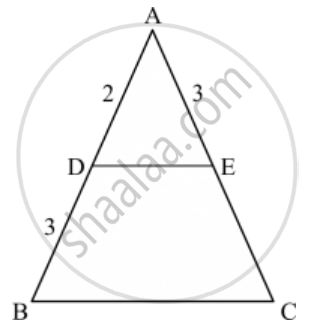Advertisement Remove all ads

# In the Given Figure, If ∠Ade = ∠Abc, Then Ce =(A) 2 (B) 5 (C) 9/2 (D) 3 - Mathematics

MCQ

In the given figure, if ∠ADE = ∠ABC, then CE =#### Options

• 2

• 5

• 9/2

• 3

Advertisement Remove all ads

#### Solution

Given: ∠ ADE = ∠ ABC

To find: The value of CE

Since ∠ ADE = ∠ ABC

∴ DE || BC       (Two lines are parallel if the corresponding angles formed are equal)

According to basic proportionality theorem if a line is parallel to one side of a triangle intersecting the other two sides, then it divides the two sides in the same ratio.

In ∆ABC, DE || BC

(AD)/(DB)=(AE)/(EC)

2/3=3/EC

EC=(3xx3)/2

EC =9/2

Hence we got the result C

Concept: Triangles Examples and Solutions
Is there an error in this question or solution?
Advertisement Remove all ads

#### APPEARS IN

RD Sharma Class 10 Maths
Chapter 7 Triangles
Q 34 | Page 134
Advertisement Remove all ads

#### Video TutorialsVIEW ALL 

Advertisement Remove all ads
Share
Notifications

View all notifications

Forgot password?
Course